# FFT 2D

This is an example of a 2D discrete Fourier transform, applied to greyscale images. The leftmost images are the input, the center images and rightmost images represent the magnitude and phase of the Fourier transform. Note that for the magnitude plot, the 0 frequency is in the middle, with positive to the right and negative frequencies to the left.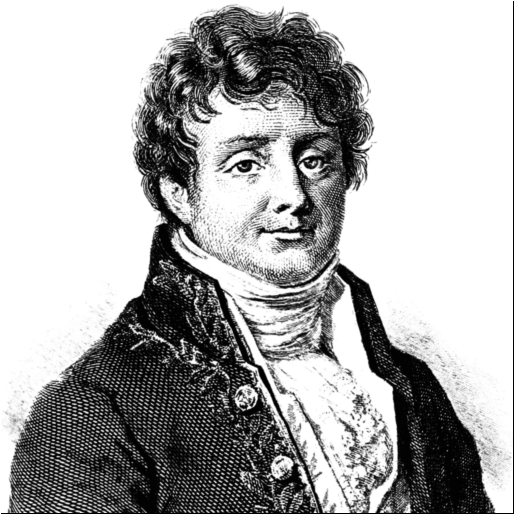Joseph Fourier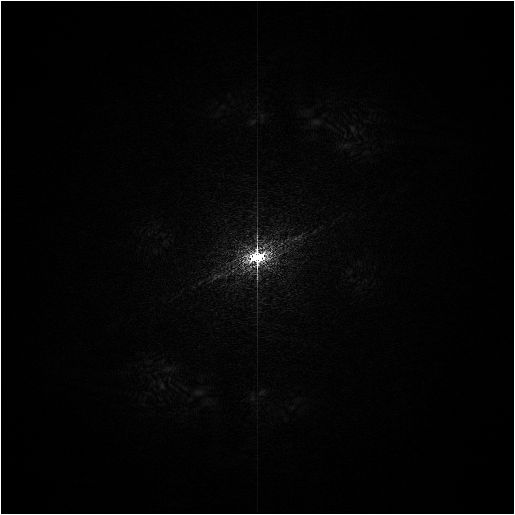Magnitude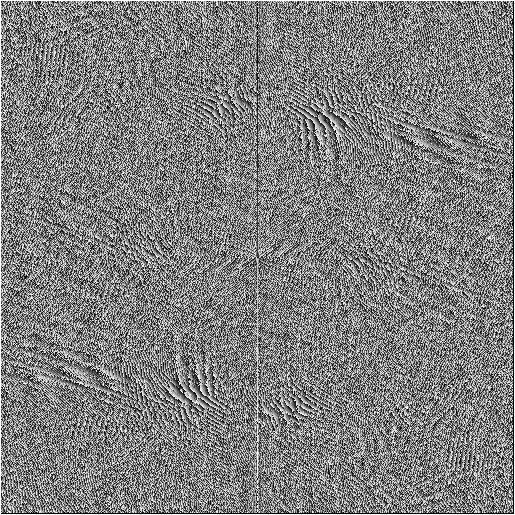Phase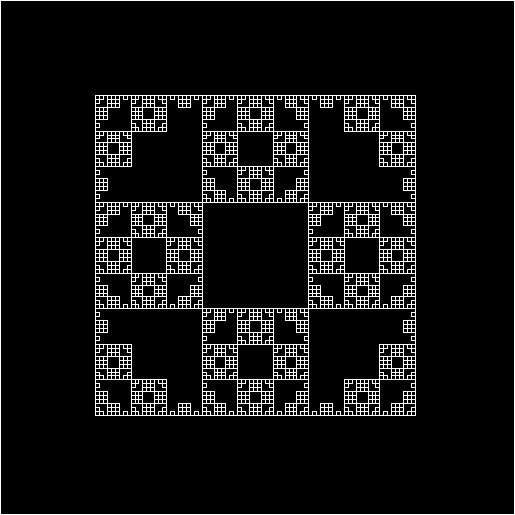Sierpinsky fr.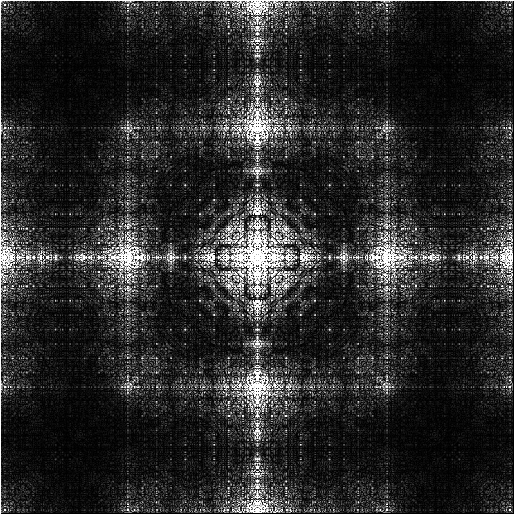Magnitude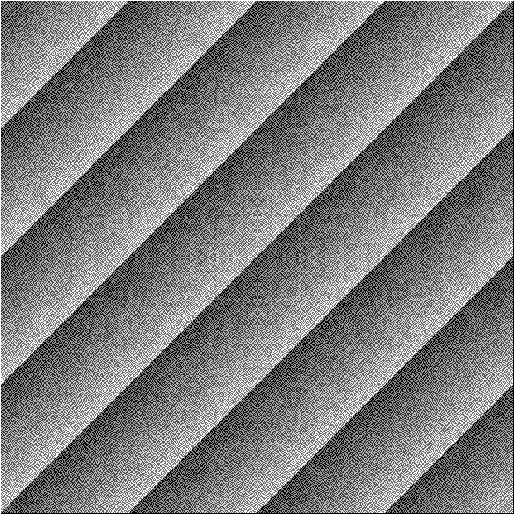Phase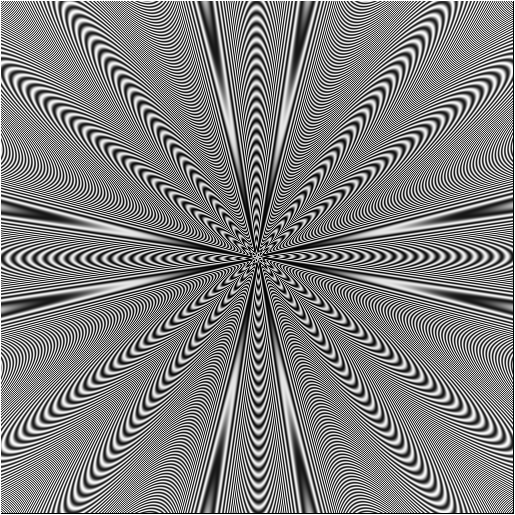Polar shape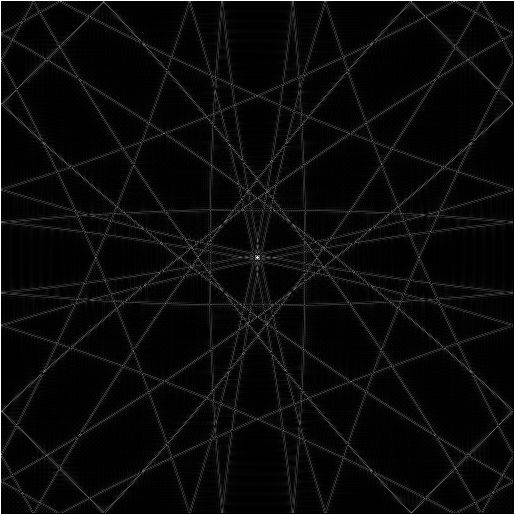Magnitude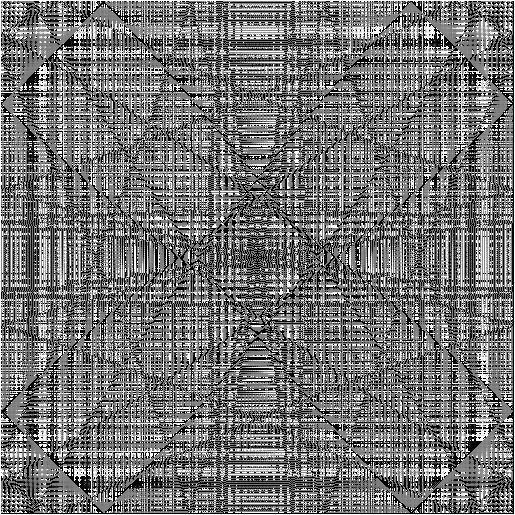PhaseAliased circle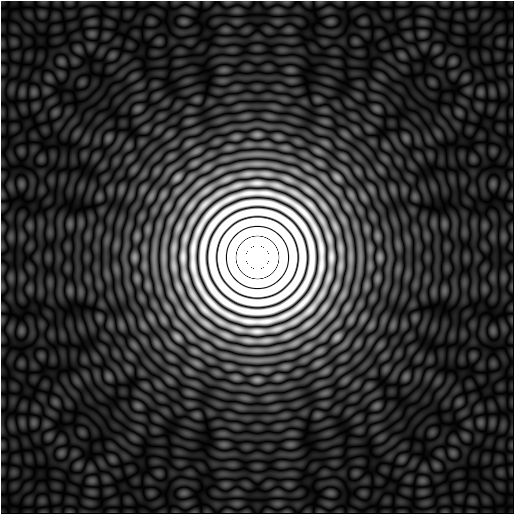Magnitude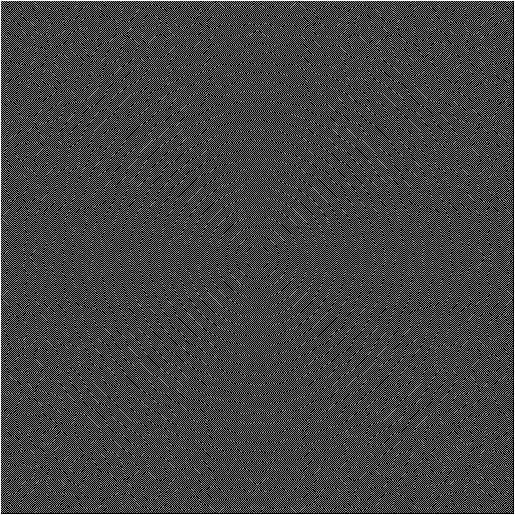Phase

## Implementation

The implementation of the 2D Fourier Transform uses a regular 1D FFT function for every row of the image, then for every column of the result. The simplest way to implement this is by transforming every row of the input, rotating the resulting data (so that columns become rows), transforming the rotated data, and then rotating it back.

Using this FFT function:

```void FFT( complex *data, int data_size );
```
I implemented the FFT2D like this:
```void FFT2D(complex *data, int w, int h)
{
// Transform every row
for (int y = 0; y < h; ++y)
FFT(&data[y * w], w);

rotate_left();

for (int y = 0; y < w; ++y)
FFT(&data[y * h], h);

rotate_right();
}
```